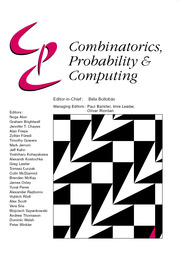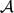Home
Hostname: page-component-59b7f5684b-hd9dq Total loading time: 0.292 Render date: 2022-09-29T22:37:32.690Z Has data issue: true Feature Flags: { "shouldUseShareProductTool": true, "shouldUseHypothesis": true, "isUnsiloEnabled": true, "useRatesEcommerce": false, "displayNetworkTab": true, "displayNetworkMapGraph": false, "useSa": true } hasContentIssue trueCombinatorics, Probability and Computing

# Manipulative Waiters with Probabilistic Intuition

Published online by Cambridge University Press:  21 December 2015

## Abstract

For positive integers n and q and a monotone graph property$\mathcal{A}$, we consider the two-player, perfect information game WC(n, q,$\mathcal{A}$), which is defined as follows. The game proceeds in rounds. In each round, the first player, called Waiter, offers the second player, called Client, q + 1 edges of the complete graph Kn which have not been offered previously. Client then chooses one of these edges which he keeps and the remaining q edges go back to Waiter. If, at the end of the game, the graph which consists of the edges chosen by Client satisfies the property$\mathcal{A}$, then Waiter is declared the winner; otherwise Client wins the game. In this paper we study such games (also known as Picker–Chooser games) for a variety of natural graph-theoretic parameters, such as the size of a largest component or the length of a longest cycle. In particular, we describe a phase transition type phenomenon which occurs when the parameter q is close to n and is reminiscent of phase transition phenomena in random graphs. Namely, we prove that if q ⩾ (1 + ϵ)n, then Client can avoid components of order cϵ−2 ln n for some absolute constant c > 0, whereas for q ⩽ (1 − ϵ)n, Waiter can force a giant, linearly sized component in Client's graph. In the second part of the paper, we prove that Waiter can force Client's graph to be pancyclic for every qcn, where c > 0 is an appropriate constant. Note that this behaviour is in stark contrast to the threshold for pancyclicity and Hamiltonicity of random graphs.

## MSC classification

Type
Paper
Information
Combinatorics, Probability and Computing , November 2016 , pp. 823 - 849

## Access options

Get access to the full version of this content by using one of the access options below. (Log in options will check for institutional or personal access. Content may require purchase if you do not have access.)

## References

 Ajtai, M., Komlós, J. and Szemerédi, E. (1985) First occurrence of Hamilton cycles in random graphs. In Cycles in Graphs, Vol. 115 of North-Holland Mathematics Studies, North-Holland, pp. 173178.Google Scholar
 Antoniuk, S., Grosu, C. and Narins, L. On the connectivity Waiter–Client game. arXiv:1510.05852 Google Scholar
 Beck, J. (1982) Remarks on positional games. Acta Math. Acad. Sci. Hungar. 40 6571.CrossRefGoogle Scholar
 Beck, J. (1985) Random graphs and positional games on the complete graph. Ann. Discrete Math. 28 713.Google Scholar
 Beck, J. (2002) Positional games and the second moment method. Combinatorica 22 169216.CrossRefGoogle Scholar
 Beck, J. (2008) Combinatorial Games: Tic-Tac-Toe Theory , Vol. 14 of Encyclopedia of Mathematics and its Applications, Cambridge University Press.Google Scholar
 Bednarska-Bzdega, M. (2013) On weight function methods in Chooser–Picker games. Theoret. Comput. Sci. 475 2133.CrossRefGoogle Scholar
 Bednarska-Bzdega, M., Hefetz, D. and Łuczak, T. Picker–Chooser fixed graph games. J. Combin. Theory Ser. B, to appear. arXiv:1402.7308 Google Scholar
 Bednarska, M. and Łuczak, T. (2001) Biased positional games and the phase transition. Random Struct. Alg. 18 141152.3.0.CO;2-W>CrossRefGoogle Scholar
 Bollobás, B. (1984) The evolution of sparse graphs. In Graph Theory and Combinatorics, Academic Press, pp. 3557.Google Scholar
 Bollobás, B. (2001) Random Graphs, second edition, Cambridge University Press.CrossRefGoogle Scholar
 Chvátal, V. and Erdős, P. (1978) Biased positional games. Ann. Discrete Math. 2 221228.CrossRefGoogle Scholar
 Cooper, C. and Frieze, A. M. (1990) Pancyclic random graphs. In Random Graphs '87, Wiley, pp. 2939.Google Scholar
 Csernenszky, A. (2010) The Picker–Chooser diameter game. Theoret. Comput. Sci. 411 37573762.CrossRefGoogle Scholar
 Csernenszky, A., Mándity, C. I. and Pluhár, A. (2009) On Chooser–Picker positional games. Discrete Math. 309 51415146.CrossRefGoogle Scholar
 Erdős, P. and Rényi, A. (1960) On the evolution of random graphs. Magyar Tud. Akad. Mat. Kutató Int. Közl. 5 1761.Google Scholar
 Erdős, P. and Selfridge, J. L. (1973) On a combinatorial game. J. Combin. Theory Ser. A 14 298301.CrossRefGoogle Scholar
 Ferber, A., Krivelevich, M. and Naves, H. (2015) Generating random graphs in biased Maker-Breaker games, Random Struct. Alg., 47, 615634.CrossRefGoogle Scholar
 Friedman, J. and Pippenger, N. (1987) Expanding graphs contain all small trees. Combinatorica 7 7176.CrossRefGoogle Scholar
 Gebauer, H. and Szabó, T. (2009) Asymptotic random graph intuition for the biased connectivity game. Random Struct. Alg. 35 431443.CrossRefGoogle Scholar
 Hales, A. W. and Jewett, R. I. (1963) Regularity and positional games. Trans. Amer. Math. Soc. 106 222229.CrossRefGoogle Scholar
 Hefetz, D., Krivelevich, M., Stojaković, M. and Szabó, T. (2010) Avoider–Enforcer: The rules of the game. J. Combin. Theory Ser. A 117 152163.CrossRefGoogle Scholar
 Hefetz, D., Krivelevich, M., Stojaković, M. and Szabó, T. (2014) Positional Games, Birkhäuser.CrossRefGoogle Scholar
 Hefetz, D., Krivelevich, M. and Szabó, T. (2007) Avoider–Enforcer games. J. Combin. Theory Ser. A 114 840853.CrossRefGoogle Scholar
 Janson, S., Łuczak, T. and Ruciński, A. (2000) Random Graphs, Wiley.CrossRefGoogle Scholar
 Komlós, J. and Szemerédi, E. (1983) Limit distribution for the existence of Hamiltonian cycles in a random graph. Discrete Math. 43 5563.CrossRefGoogle Scholar
 Krivelevich, M. (2011) The critical bias for the Hamiltonicity game is (1 + o(1))n/ lnn. J. Amer. Math. Soc. 24 125131.CrossRefGoogle Scholar
 Krivelevich, M. and Sudakov, B. (2013) The phase transition in random graphs: A simple proof. Random Struct. Alg. 43 131138.CrossRefGoogle Scholar
 Krivelevich, M. and Szabó, T. (2008) Biased positional games and small hypergraphs with large covers. Electron. J. Combin. 15 R70.Google Scholar
 Lehman, A. (1964) A solution of the Shannon switching game. J. Soc. Indust. Appl. Math. 12 687725.CrossRefGoogle Scholar
 Łuczak, T. (1991) Cycles in random graphs. Discrete Math. 98 231236.CrossRefGoogle Scholar
 Pósa, L. (1976) Hamiltonian circuits in random graphs. Discrete Math. 14 359364.CrossRefGoogle Scholar
 West, D. B. (2001) Introduction to Graph Theory, second edition, Prentice Hall.Google Scholar
6
Cited by

# Save article to Kindle

To save this article to your Kindle, first ensure coreplatform@cambridge.org is added to your Approved Personal Document E-mail List under your Personal Document Settings on the Manage Your Content and Devices page of your Amazon account. Then enter the ‘name’ part of your Kindle email address below. Find out more about saving to your Kindle.

Note you can select to save to either the @free.kindle.com or @kindle.com variations. ‘@free.kindle.com’ emails are free but can only be saved to your device when it is connected to wi-fi. ‘@kindle.com’ emails can be delivered even when you are not connected to wi-fi, but note that service fees apply.

Find out more about the Kindle Personal Document Service.

Manipulative Waiters with Probabilistic Intuition
Available formats
×

# Save article to Dropbox

To save this article to your Dropbox account, please select one or more formats and confirm that you agree to abide by our usage policies. If this is the first time you used this feature, you will be asked to authorise Cambridge Core to connect with your Dropbox account. Find out more about saving content to Dropbox.

Manipulative Waiters with Probabilistic Intuition
Available formats
×

# Save article to Google Drive

To save this article to your Google Drive account, please select one or more formats and confirm that you agree to abide by our usage policies. If this is the first time you used this feature, you will be asked to authorise Cambridge Core to connect with your Google Drive account. Find out more about saving content to Google Drive.

Manipulative Waiters with Probabilistic Intuition
Available formats
×
×